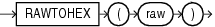## RAWTOHEX

SyntaxDescription of the illustration rawtohex.eps

Purpose

`RAWTOHEX` converts `raw` to a character value containing its hexadecimal representation.

As a SQL built-in function, `RAWTOHEX` accepts an argument of any scalar data type other than `LONG`, `LONG` `RAW`, `CLOB`, `NCLOB`, `BLOB`, or `BFILE`. If the argument is of a data type other than `RAW`, then this function converts the argument value, which is represented using some number of data bytes, into a `RAW` value with the same number of data bytes. The data itself is not modified in any way, but the data type is recast to a `RAW` data type.

This function returns a `VARCHAR2` value with the hexadecimal representation of bytes that make up the value of `raw`. Each byte is represented by two hexadecimal digits.

Note:

`RAWTOHEX` functions differently when used as a PL/SQL built-in function. Refer to Oracle Database Development Guide for more information.

Appendix C in Oracle Database Globalization Support Guide for the collation derivation rules, which define the collation assigned to the character return value of `RAWTOHEX`

Examples

The following hypothetical example returns the hexadecimal equivalent of a `RAW` column value:

```SELECT RAWTOHEX(raw_column) "Graphics"
FROM graphics;

Graphics
--------
7D  ```